Splitting Criteria

Subsections:

When you specify entropy or the Gini statistic as the splitting criterion, the value of the split is judged by the decrease in the specified criterion. Thus, the criterion for the original leaf is computed, as is the criterion for the final, split leaf. The per-variable split and then the variable on which to split are chosen based on the gain.

When you specify FastCHAID as the splitting criterion, splitting is based on the Kolmogorov-Smirnov distance of the variables.

Entropy Splitting Criterion

The entropy is related to the amount of information that a split contains. The entropy of a single leafis given by the equation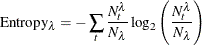where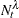is the number of observations with the target level t on leafand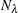is the number of observations on the leaf (Hastie, Tibshirani, and Friedman, 2001; Quinlan, 1993).

When a leaf is split, the total entropy is then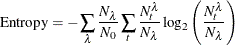where
is the number of observations on the original unsplit leaf.

Gini Splitting Criterion

Split Gini is similar to split entropy. First, the per-leaf Gini statistic or index is given by Hastie, Tibshirani, and Friedman (2001) as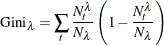When split, the Gini statistic is then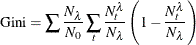Kolmogorov-Smirnov (FastCHAID) Splitting Criterion

The Kolmogorov-Smirnov (K-S) distance is the maximum distance between the cumulative distribution functions (CDFs) of two or more target levels (Friedman, 1977; Rokach and Maimon, 2008; Utgoff and Clouse, 1996). To create a meaningful CDF for nominal inputs, nominal target levels are ordered first by the level that is specified in the EVENT= option in the PROC HPSPLIT statement (if specified) and then by the other levels in internal order.

After the CDFs have been created, the maximum K-S distance is given by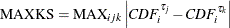where i is an interval variable bin or an explanatory variable level,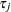is the jth target level, and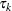is the kth target level.

At each step of determining each variable’s split, the maximum K-S distance is computed, resulting in a single split. The splitting continues recursively until the value specified in the MAXBRANCH= option has been reached.

After each variable’s split has been determined, the variable that has the lowest p-value is chosen as the variable on which to split. Because this operation is similar to another established tree algorithm (Kass, 1980; Soman, Diwakar, and Ajay, 2010), this overall criterion is called FastCHAID.

Chi-Square Splitting Criterion

The chi-square criterion uses the sum of squares of the two-way contingency table that is formed by an input variable’s levels and the target levels.

For C children of the split, the sum is equal to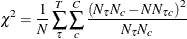where N is the number of observations under consideration, T is the number of target levels,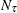is the number of observations that have target level,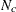is the number of observations assigned to child c, and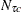is the number of observations that have target leveland are assigned to child c.

After splitting each variable, the variable that has the lowest p-value is chosen as the variable on which to split.

Variance Splitting Criterion

The variance criterion chooses the split that has the maximum reduction in variance. For some leaf, variance is calculated as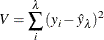So the reduction in variance then becomes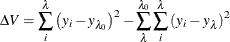where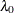is the leaf being split andare the leaves after the split.

This is a type of change in purity criterion.

F Test Splitting Criterion

The F test criterion uses the F sum to choose the best split for a variable: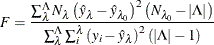After splitting each variable, the variable that has the lowest F test p-value is chosen as the variable on which to split. The p-value is the probability that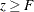, where z is a random variable from an F distribution that has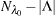,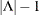degrees of freedom. Because the value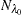is the sum of weights on the unsplit leaf, this is not a strictly correct degrees of freedom.